# NCERT Solutions for Class 10 Maths Chapter 1 Real Numbers Ex 1.4

NCERT Maths Solutions for Ex 1.4 Class 10 Real Numbers is the perfect guide to boost up your preparation during CBSE 10th Class Maths Examination.

NCERT Solutions for Class 10 Maths Chapter 1 Real Numbers Ex 1.4 are part of . Here are we have given Chapter 1 Real Numbers Class 10 NCERT Solutions Ex 1.4.

 Board CBSE Textbook NCERT Class Class 10 Subject Maths Chapter Chapter 1 Chapter Name Real Numbers Exercise Ex 1.4 Number of Questions Solved 3 Category NCERT Solutions

## NCERT Solutions for Class 10 Maths Chapter 1 Real Numbers Ex 1.4

Page No: 17

Question 1
Without actually performing the long division, state whether the following rational numbers will have a terminating decimal expansion or a non-terminating repeating decimal expansion:
(i) 13/3125
(ii) 17/8
(iii) 64/455
(iv) 15/1600
(v) 29/343
(vi) 23/23 × 52
(vii) 129/22 × 57 × 75
(viii) 6/15
(ix) 35/50
(x) 77/210

Solution: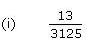3125 = 55
The denominator is of the form 5m.8 = 23
The denominator is of the form 2m.455 = 5 x 7 x 13
Since the denominator is not in the form 2m x 5n, and it also contains 7 and 13 as its factors, its decimal expansion will be non-terminating repeating.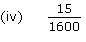1600 = 26 × 52
The denominator is of the form 2m x 5n.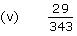343 = 73
Since the denominator is not in the form 2m x 5n, and it has 7 as its factor,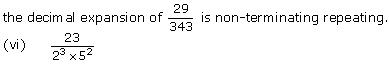The denominator is of the form 2m x 5n. Hence, the decimal expansion of is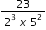terminating.

(vii)Since the denominator is not of the form 2m  5n, and it also has 7 as itsThe denominator is of the form 5n.
10 = 2 x 5
The denominator is of the form 2m x 5n.30 = 2 x 3 x 5
Since the denominator is not of the form 2m × 5n, and it also has 3 as its factors,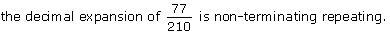Concept Insight: The concept used in this problem is that The decimal expansion of rational number p/q where p and q are coprime numbers, terminates if and only if the prime factorization of q is of the form 2n5m, where n and m are non negative integers. Do not forget that 0 is also a non negative integer so n or m can take value 0.
Generally, mistake is committed in identifying terminating decimals when either of the two prime numbers  2 or 5 is appearing in the prime factorization.

Page No: 18

Question 2
Write down the decimal expansions of those rational numbers in Question 1 above which have terminating decimal expansions.

Solution: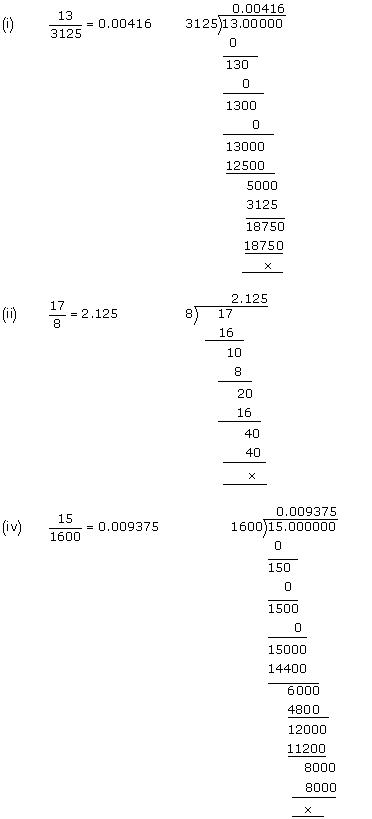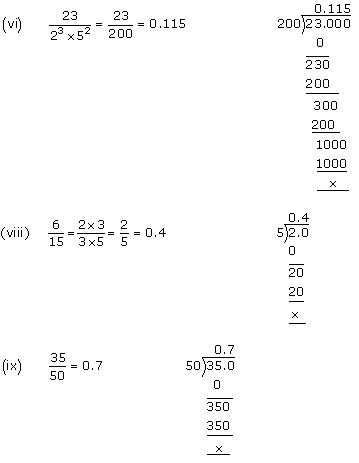Concept Insight:
This is based on performing the long division and expressing the rational number in the decimal form learned in lower classes.

Question 3
The following real numbers have decimal expansions as given below. In each case, decide whether they are rational or not. If they are rational, and of the form p, q you say about the prime factors of q?
(i) 43.123456789
(ii) 0.120120012000120000…
(iii) 43.123456789

Solution:
(i) 43.123456789
Since this number has a terminating decimal expansion, it is a rational number of the form  p\q and q is of the form 2m x 5n,
i.e., the prime factors of q will be either 2 or 5 or both.

(ii) 0.120120012000120000…
The decimal expansion is neither terminating nor recurring. Therefore, the given number is an irrational number.

(iii) 43.123456789
Since the decimal expansion is non-terminating recurring, the given number is a rational number of the form p/q and q is not of the form 2m x 5n  i.e., the prime factors of q will also have a factor other than 2 or 5.

Concept Insight: The concept used in this problem is that, If the decimal expansion of rational number p\q, [where p and q are coprime numbers] terminates, then prime factorization of q is of the form 2n5m, where n and m are non negative integers.

We hope the NCERT Solutions for Class 10 Maths Chapter 1 Real Numbers Ex 1.4 help you. If you have any query regarding NCERT Solutions for Class 10 Maths Chapter 1 Real Numbers Ex 1.4, drop a comment below and we will get back to you at the earliest.